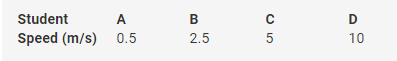# Please give me all practical based question in class 9 in science

Please give me all practical based question in class 9 in science.[Please give the question and if possible answers directly, don’t say to see the question papers]

1. What happens when solutions of sodium sulphate and barium chloride are mixed? Give the reaction.
(2)
When solutions of sodium sulphate and barium chloride are mixed, a white-coloured precipitate of barium sulphate is formed along with sodium chloride solution.
Na2SO4 + BaCl2 → 2NaCl + BaSO4
2. In an experiment, 18.5 g of copper sulphate reacted with 11.0 g of sodium hydroxide to form 10.0 g of copper hydroxide and 19.5 g of sodium sulphate. Which law of chemical combination is illustrated by this data? Give reason for your choice. (2)
In an experiment, 18.5 g of copper sulphate reacted with 11.0 g of sodium hydroxide to form 10.0 g of copper hydroxide and 19.5 g of sodium sulphate.
CuSO4(18.5 g) + NaOH(11.0 g) → Cu(OH)(10.0 g) 2 + NaSO4(19.5 g)
From the given data, we get
Total mass of the reactants = (18.5 g + 11.0 g) = 29.5 g
Total mass of the products = (10.0 g + 19.5 g) = 29.5 g
Hence, the law of conservation of mass is valid here.
It states that mass can neither be created nor destroyed in a chemical reaction.
Total mass of the reactant is equal to the total mass of the product.
3. While verifying the laws of reflection of sound, a student measured the angle between the incident sound wave and reflected sound wave as 100°. What will be the angle of reflection?
According to the law of reflection of sound, the angle of the incident sound wave is equal to the angle of the reflected sound wave.
® Ði = Ðr
Given that i + r = 100
or 2i = 100
∴ Ði = 50 =Ðr
4. A pulse was created in a stretched string of length of 5 m by four students A, B, C and D. They observed that the pulse returned after reflection at the point of creation 5 times in 10 seconds and calculated the speed as given in the table below: (2)Which student has reported the speed correctly?
Speed is distance divided by time.
Distance is the length of the stretched string, d = 5 m
Time is the time taken by the pulse for 1 to-and-fro motion.
Here, the pulse travels 5 to-and-fro motions in 10 seconds.
Therefore, the time taken is 10/5 = 2 s
Hence, the speed of the pulse is
Hence, student B reported the speed correctly.
5. You are given a mixture of carbon disulphide, benzene and water. How will the layers form and why? Suggest a method to separate them.
A mixture of more than two immiscible liquids is separated by using a separating funnel. When a mixture of carbon disulphide, benzene and water is put in a separating funnel, it separates into three layers. Carbon disulphide (being heavier than water) forms the bottom layer, water forms the middle layer and benzene (being the lightest) forms the top layer. On opening the stop-cock, carbon disulphide will run out first followed by water and then benzene.
6. There are two nails A and B. Nail A is sharp and nail B is blunt. The area of nail A is
0.1 m2 and that of nail B is 1.5 m2. These nails are to be hammered into a wooden plank. Which nail will be hammered more easily if the force applied is 200 N to hammer both nails? Explain why. Calculate the pressure required to hammer each nail. (2)
Nail A is the pointed one, so it has a surface area which is less than nail B. Thus, nail A will move inside the wooden plank more easily than nail B.
Area of nail A =Aa= 0.1 m2
Area of nail B=Ab= 1.5 m2
Force applied on both nails = 200 N
Pressure for nail A =PA= F = 200 = 2000 Pa
Aa0.1
Pressure for nail B =PB= F = 200 = 133.3 Pa
Ab1.5
Hence,
PA>PB (less the surface area, more is the pressure applied to that object)
7. On which principle is the working of a stethoscope based? State a one-word term for the text ‘hall giving many echoes when sound is incident on its ceiling or wall’.
A stethoscope works on the principle of multiple reflections.
The one-word term reverberation can be used to explain the text.
(2)
8. Which of the following will show Tyndall effect?
a) Salt solution
b) Milk
c) Copper sulphate solution
d) Starch solution
Milk and starch solution are colloids and hence will show Tyndall effect.
Copper sulphate solution and salt solution will not show Tyndall effect.
(2)